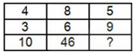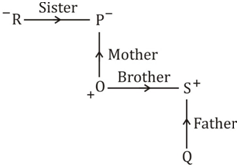# SSC Reasoning Ability Practice Questions (Day-76)

Dear Aspirants, The SSC Exams are going on at national level. Millions of candidates have eagerly prepared for the ongoing SSC exams such as CGL, CHSL, MTS, CPO, JE etc. SSC exams are usually conducted by the Staff Selection Commission to recruit the eligible candidates for various Central government departments. Mostly the selection process for all the SSC exams consists of the single or double phase of written examination based on the examination. Due to that, the written exam consisted of a certain syllabus and exam pattern. By following those regulations, the written exam has been conducted through an online mode. Based on the latest updated exam pattern and syllabus, here we have drafted the Reasoning Ability questions on a daily basis. So aspirants start your practice session with us and mould your skill in a perfect shape.

Start Quiz

1) If ‘A + B’ means ‘A is brother of B’, ‘A – B’ means ‘A is sister of B’, ‘A * B’ means ‘A is father of B’, ‘A ÷ B’ means ‘A is mother of B’, then which of thefollowing option signifies that P is grandmother of Q?

(a) R – P * O – S – Q

(b) R – P ÷ O + S * Q

(c) R + P * O + S * Q

(d) R – P * O + S ÷ Q

Direction (2-3): In the following question, select the word which cannot be formed using the letters of the given word.

(b) RATION

(c) NATIONAL

(d) TRAIN

3) TREASURER

(a) SURE

(b) TREAT

(c) REST

(d) TEASE

4) In a certain code language, “pink” is written as “green”, “green” is written as “yellow”, “yellow” is written as “red”, “red” is written as “white” and”white” is written as “indigo”, then in the same code language what is the colour of blood?

(a) white

(b) red

(c) green

(d) yellow

5) In a certain code language, “BRAIN” is written as “PGCPD” and “STOLE” is written as “GJQRU”. How is “ZURIC” written in that code language?

(a) DIPVY

(b) EGTSB

(c) DKUVB

(d) EKTWB

6) In the following question, correct the equation by interchanging two signs.

18 ÷ 3 + 9 – 6 × 3 = 15

(a) – and ×

(b) + and –

(c) ÷ and –

(d) + and ÷

7) If “P” denotes “added to”, “Q” denotes “subtracted from”, “R” denotes “divided by” and “S” denotes “multiplied by”, then which of the followingequation is true?

(a) 18 S 12 R 4 P 5 Q 6 = 65

(b) 4 P 57 R 3 Q 7 S 3 = – 2

(c) 6 P 7 Q 12 R 6 S 3 = 7

(d) 29 Q 28 R 2 P 3 S 2 = 10

8) If 436 = 3, 783 = 8 and 654 = 5, then 896 = ?

(a) 5

(b) 8

(c) 13

(d) 6

9) If 32 + 21 = 2809 and 27 + 15 = 1764, then 31 + 13 = ?

(a) 1444

(b) 2116

(c) 1936

(d) 2304

Direction (10): In the following question, select the number which can be placed at the sign of question mark (?) from the given alternatives.

10)(a) 43

(b) 45

(c) 47

(d) 41∴ P is grandmother of Q.

∴ R – P ÷ O + S * Q is the correct relation.

‘NATIONAL’; There is no double ‘N’ in the given word.

‘TREAT’ ; There is no double ‘T’ in the given word.

Colour of blood is red and Red in written as white, so, white is the answer.18 ÷ 3 + 9 – 6  × 3 = 15

⇒ 18 ÷ 3 – 9 + 6 × 3 = 15

⇒ 6 – 9 + 18 = 15

⇒ 24 – 9 = 15

⇒ 15 = 15

6 P 7 Q 12 R 6 S 3 = 7

⇒ 6 + 7 – 12 ÷ 6 × 3 = 7

⇒ 6 + 7 – 2 × 3 = 7

⇒ 6 + 7 – 6 = 7

⇒ 7 = 7

(4 + 3 + 6) – 10 = 3

(7 + 8 + 3) – 10 = 8

(6 + 5 + 4) – 10 = 5

(8 + 9 + 6) – 10 = 13

(32 + 21)2 = 2809

(27 + 15)2 = 1764

(31 + 13)2 = 1936

(4 × 3) – 2 = 10

(8 × 6) – 2 = 46

(5 × 9) – 2 = 43

 Check Here to View SSC CGL / CHSL 2021 Reasoning Ability Questions Day – 75 Day – 74 Day – 73